Matrix Formulation of the DFT Next  |  Prev  |  Up  |  Top  |  Index  |  JOS Index  |  JOS Pubs  |  JOS Home  |  Search

## Matrix Formulation of the DFT

The DFT can be formulated as a complex matrix multiply, as we show in this section. (This section can be omitted without affecting what follows.) For basic definitions regarding matrices, see Appendix H.

The DFT consists of inner products of the input signal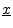with sampled complex sinusoidal sections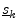:By collecting the DFT output samples into a column vector, we have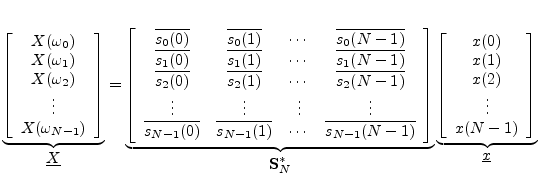or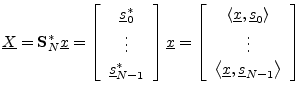where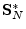denotes the DFT matrix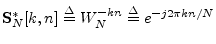, i.e.,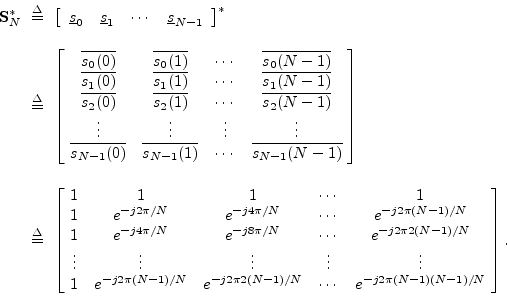The notation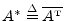denotes the Hermitian transpose of the complex matrix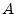(transposition and complex conjugation).

Note that the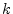th column of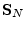is theth DFT sinusoid, so that theth row of the DFT matrixis the complex-conjugate of theth DFT sinusoid. Therefore, multiplying the DFT matrix times a signal vectorproduces a column-vector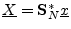in which theth element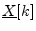is the inner product of theth DFT sinusoid with, or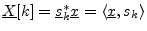, as expected.

Computation of the DFT matrix in Matlab is illustrated in §I.4.3.

The inverse DFT matrix is simply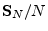. That is, we can perform the inverse DFT operation as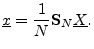(6.1)

Since the forward DFT is, substitutingfrom Eq. (6.1) into the forward DFT leads quickly to the conclusion that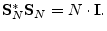(6.2)

This equation succinctly states that the columns ofare orthogonal, which, of course, we already knew. I.e.,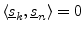for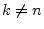, and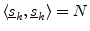: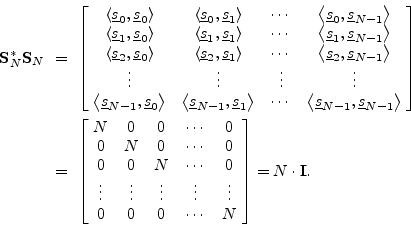The normalized DFT matrix is given byand the corresponding normalized inverse DFT matrix is simply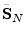, so that Eq. (6.2) becomes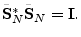This implies that the columns ofare orthonormal. Such a complex matrix is said to be unitary.

When a real matrix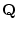satisfies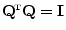, thenis said to be orthogonal. Unitary'' is the generalization of orthogonal'' to complex matrices.

Next  |  Prev  |  Up  |  Top  |  Index  |  JOS Index  |  JOS Pubs  |  JOS Home  |  Search

[How to cite this work] [Order a printed hardcopy]